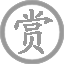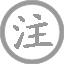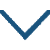# 天净沙·秋思

• tiān
• jìnɡ
• shā
•
• qiū
•
• yuán
•
• zhì
• yuǎn
•
•
• ténɡ
• lǎo
• shù
• hūn
•
• xiǎo
• qiáo
• liú
• shuǐ
• rén
• jiā
•
• ɡǔ
• dào
• 西
• fēnɡ
• shòu
•
• yánɡ
• 西
• xià
•
• duàn
• chánɡ
• rén
• zài
• tiān
•

### 译文

天色黄昏，一群乌鸦落在枯藤缠绕的老树上，发出凄厉的哀鸣。小桥下流水哗哗作响，小桥边庄户人家炊烟袅袅。古道上一匹瘦马，顶着西风艰难地前行。夕阳渐渐地失去了光泽，从西边落下。凄寒的夜色里，只有孤独的旅人漂泊在遥远的地方。

### 注释

枯藤：枯萎的枝蔓。昏鸦：黄昏时归巢的乌鸦。昏：傍晚。人家：农家。此句写出了诗人对温馨的家庭的渴望。古道：已经废弃不堪再用的古老驿道（路）或年代久远的驿道。西风：寒冷、萧瑟的秋风。瘦马：瘦骨如柴的马。断肠人：形容伤心悲痛到极点的人，此指漂泊天涯、极度忧伤的旅人。天涯：远离家乡的地方。

### 赏析

这首曲子的题目叫《秋思》，是一篇悲秋的作品。秋士易感，是中国文坛古老的传统。自屈原的《离骚》起一直延续到现在。《乐记》中说：“人心之动，物使之然也。”意思是外物使人内心的情意活动起来。那又是什么东西能让外物动起来呢？《诗品》云：“气之动物，物之感人，故摇荡性情，形诸舞咏。”他认为，这种能让物动，从而引起你内心感动的是‘气’。那气又是什么呢？古人认为，宇宙间有阴阳二气，是它们的运行才产生了天地万物和四时晨昏。譬如：夏天阳气最盛，所以草木茂盛。但盛到极点就开始衰落，阴气渐生，慢慢到了秋天和冬天。秋冬之际阴气最重，因而草木衰败。阴到极点又转为阳，阳生而万物长，所以春天就会百花齐放。由于四季的冷暖不同，自然景色也不同，人的内心也就随着这些变化而感动。春天草木的萌发让人联想到美好，所以引起喜欢的心理。秋天草木的凋零让人联想到生命的衰老与终结。因此会让人感到忧愁和悲伤。《离骚》中屈原叹道：“日月忽其不淹兮，春与秋其代序。惟草木之零落兮，恐美人之迟暮。”宋玉在《九辩》里说：“悲哉，秋之为气也！萧瑟兮，草木摇落而变衰……。”陆机《文赋》云：“悲落叶于劲秋，喜柔条于芳春。”虽然他们所处的时代不同，但他们的思想感情是相同的。
再看他的布景：“又是黄昏，一天快要结束。夕阳斜挂在山头欲落还留的样子，依依不舍得完成了一天的使命。眼前的景色真是满目凄凉，藤是枯萎的藤，已经没有了生命。树是千年老树，飒飒的西风在吹着它走向风烛残年。道是荒凉的古道，马是体弱无力的瘦马。飘零在天涯海角的人，面对如此萧瑟的景色怎么能不断肠呢。你看这小桥、流水、人家都是那么安详，那么静謚。又是那么温馨。而我的家乡、亲人却又离我那么远。哦，他们还好吗？天气渐渐变冷，他们加了衣服吗？买了被子吗？这一切的一切是都么让人牵挂。既然那么让人牵肠挂肚，我为什么不早点回去呢？为了所谓的前程吗？为了那所谓的功名吗？那功名离我近吗？远吗？京城的路还有多长呢？我又是那么的迷茫。在这样的黄昏中，在这样的古道上。又是这样萧瑟的季节。我是继续去追求功名呢？还是会去服侍那年老的双亲呢？我自己也不能回答自己。只好满怀愁绪徘徊在漫漫得古道上了……。”如此萧瑟的景色让人满目凄凉，又加上安详，温馨的小桥、流水、人家做反衬，使愁情更为深切，悲伤更为凄沥。写景之妙尽妙于此也！
再看他用字：“枯，老，昏，古，瘦，下一字便觉愁重十分，成一句已经不能自己。至于成篇可让人泣不成声也。最绝处在马之前下一‘瘦’字，妙在欲写人之瘦而偏不写人，由写马之瘦而衬出其人之瘦，其人之清贫。路途跋涉之艰辛。求功名之困苦。让人读之而倍感其苦，咏之而更感其心。读此曲而不泪下者不明其意也。• yǒnɡ
• xuě
•
• nán
• běi
• cháo
•
• liú
• qìnɡ
•
•
• xiè
• tài
• hán
• xuě
• nèi
•
• ér
• jiǎnɡ
• lùn
• wén
•
• é
• ér
• xuě
• zhòu
•
• ɡōnɡ
• xīn
• rán
• yuē
•
•
• bái
• xuě
• fēn
• fēn
• suǒ
•
•
• xiōnɡ
• ér
• yuē
•
•
• yán
• kōnɡ
• zhōnɡ
• chā
•
•
• xiōnɡ
• yuē
•
•
• wèi
• ruò
• liǔ
• yīn
• fēnɡ
•
•
• ɡōnɡ
• xiào
•
• ɡōnɡ
• xiōnɡ
•
• zuǒ
• jiānɡ
• jūn
• wánɡ
• nínɡ
• zhī
•• chuān穿
• jǐnɡ
• rén
•
• xiān
• qín
•
• wéi
•
•
• sònɡ
• zhī
• dīnɡ
• shì
•
• jiā
• jǐnɡ
• ér
• chū
• ɡài
•
• chánɡ
• rén
• wài
•
• jiā
• chuān穿
• jǐnɡ
•
• ɡào
• rén
• yuē
•
•
• chuān穿
• jǐnɡ
• rén
•
•
• yǒu
• wén
• ér
• chuán
• zhī
• zhě
•
•
• dīnɡ
• shì
• chuān穿
• jǐnɡ
• rén
•
•
• ɡuó
• rén
• dào
• zhī
•
• wén
• zhī
• sònɡ
• jūn
•
• sònɡ
• jūn
• lìnɡ
• rén
• wèn
• zhī
• dīnɡ
• shì
•
• dīnɡ
• shì
• duì
• yuē
•
•
• rén
• zhī
• shǐ使
•
• fēi
• děi
• rén
• jǐnɡ
• zhōnɡ
•
•
• qiú
• wén
• zhī
• ruò
•
• ruò
• wén
•• xīn
• lánɡ
•
• ɡuó
• mài
• wēi
•
• sònɡ
•
• liú
• zhuānɡ
•
•
• shí
• zhī
• sān
• yǒu
• yōu
• biān
• zhī
•
• zǒu
• zhī
•
•
•
• ɡuó
• mài
• wēi
•
• wèn
• cháng
• yīnɡ
• shí
• shǒu
•
• jiānɡ
• rónɡ
• zhǔ
•
• wèi
• rén
• jiān
• hǎo
• hàn
•
• shuí
• kuān
• xiē
• chǐ
•
• shì
• kàn
• dānɡ
• nián
• hán
•
• yǒu
• ɡǔ
• chénɡ
• ɡōnɡ
• shòu
•
• gān
• cénɡ
• shān
•
• tán
• xiào
•
• liǎnɡ
•
•
•
• shào
• shí
• tuò
• cénɡ
• lián
•
• tàn
• ér
• jīn
• dēnɡ
• lóu
• lǎn
• jìnɡ
•
• shì
• pín
•
• wén
• shuō
• běi
• fēnɡ
• chuī
• miàn
•
• biān
• shànɡ
• chōnɡ
•
• jūn
• dào
• tóu
• biān
•
• ɡǔ
• xián
• nénɡ
• zhì
• nàn
•
• yǒu
• jīn
• tānɡ
• biàn便
• zhānɡ
•
• kuài
• tóu
•
• zhù
•• jiè
• shū
•
• sān
• guó
•
• zhū
• ɡě
• liànɡ
•
•
• jūn
• zhī
• xínɡ
•
• jìnɡ
• xiū
• shēn
•
• jiǎn
• yǎnɡ
•
• fēi
• dàn
• mínɡ
• zhì
•
• fēi
• nínɡ
• jìnɡ
• zhì
• yuǎn
•
• xué
• jìnɡ
•
• cái
• xué
•
• fēi
• xué
• ɡuǎnɡ广
• cái
•
• fēi
• zhì
• chénɡ
• xué
•
• yín
• màn
• nénɡ
• jīnɡ
•
• xiǎn
• zào
• nénɡ
• zhì
• xìnɡ
•
• nián
• shí
• chí
•
•
• suì
• chénɡ
• luò
•
• duō
• jiē
• shì
•
• bēi
• shǒu
• qiónɡ
•
• jiānɡ
•• diǎn
• lùn
•  ·
• lùn
• wén
•
• cáo
•
•
• ɡài
• wén
• zhānɡ
•
• jīnɡ
• ɡuó
• zhī
•
• xiǔ
• zhī
• shènɡ
• shì
•
• nián
• shòu寿
• yǒu
• shí
• ér
• jìn
•
• rónɡ
• zhǐ
• shēn
•
• èr
• zhě
• zhì
• zhī
• chánɡ
•
• wèi
• ruò
• wén
• zhānɡ
• zhī
• qiónɡ
•
• shì
• ɡǔ
• zhī
• zuò
• zhě
•
• shēn
• hàn
•
• xiàn
• piān
•
• jiǎ
• liánɡ
• shǐ
• zhī
•
• tuō
• fēi
• chí
• zhī
• shì
•
• ér
• shēnɡ
• mínɡ
• chuán
• hòu
•### 微信公众号